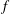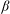Home

# Saturation of equatorial inertial instability

## Abstract

Inertial instability in parallel shear flows and circular vortices in a uniformly rotating system ($f$ -plane) redistributes absolute linear momentum or absolute angular momentum in such a way as to neutralize the instability. In previous studies we showed that, in the absence of other instabilities, at high Reynolds numbers the final equilibrium can be predicted with a simple construction based on conservation of total momentum. In this paper we continue this line of research with a study of barotropic shear flows on the equatorial${\it\beta}$ -plane. Through numerical simulations the evolution of the instability is studied in select illuminating cases: a westward flowing Gaussian jet with the flow axis exactly on the equator, a uniform shear flow and eastward and westward flowing jets that have their flow axis shifted away from the equator. In the numerical simulations it is assumed that there are no along-stream variations. This suppresses equatorial Rossby waves and barotropic shear instabilities and allows only inertial instability to develop. We investigate whether for these flows on the equatorial${\it\beta}$ -plane the final equilibrated flow can be predicted as was possible for flows on the$f$ -plane. For the Gaussian jet centred on the equator the prediction of the equilibrated flow is obvious by mere inspection of the initial momentum distribution and by assuming that momentum is mixed and homogenized to render the equilibrated flow inertially stable. For the uniform shear flow, however, due to the peculiar nature of the initial momentum distribution and the fact that the Coriolis parameter$f$ varies with latitude, it appears that, unlike in our earlier studies of flows on the$f$ -plane, additional constraints need to be considered to correctly predict the outcome of the highly nonlinear evolution of the instability. The mixing range of the linear shear flow and the value of the mixed momentum is determined numerically and this is used to predict the equilibrated flow that emerges from an eastward flowing jet that is shifted a small distance away from the equator. For shifts large enough to induce no shear at the equator the equilibrium flow can be well predicted using the simple recipe used in our earlier studies of parallel shear flows on the$f$ -plane. For the westward flowing jet shifted a very small distance from the equator, no prediction appears feasible. For modestly small shifts a prediction is possible by combining the empirical prediction for the linear shear flow with a prediction similar to what we used in our previous studies for flows on the$f$ -plane.

## References

Hide All
MathJax
MathJax is a JavaScript display engine for mathematics. For more information see http://www.mathjax.org.

# Saturation of equatorial inertial instability

## Metrics

### Full text viewsFull text views reflects the number of PDF downloads, PDFs sent to Google Drive, Dropbox and Kindle and HTML full text views.

Total number of HTML views: 0
Total number of PDF views: 0 *Loading metrics...

### Abstract viewsAbstract views reflect the number of visits to the article landing page.

Total abstract views: 0 *Loading metrics...

* Views captured on Cambridge Core between <date>. This data will be updated every 24 hours.

Usage data cannot currently be displayed.